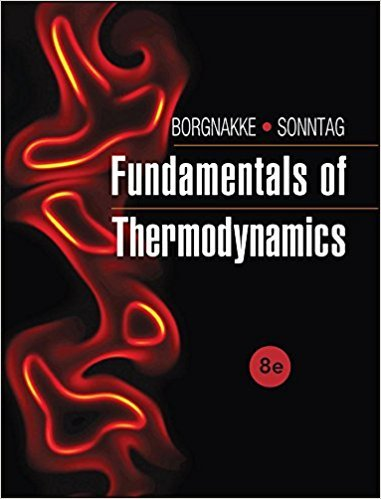×
Get Full Access to Fundamentals Of Thermodynamcs - 8 Edition - Chapter 4 - Problem 137hp
Get Full Access to Fundamentals Of Thermodynamcs - 8 Edition - Chapter 4 - Problem 137hp

×

# A nitrogen line at 300 K, 0.5 MPa, shown in Fig. P4.137,ISBN: 9781118131992 58

## Solution for problem 137HP Chapter 4

Fundamentals of Thermodynamcs | 8th Edition

• Textbook Solutions
• 2901 Step-by-step solutions solved by professors and subject experts
• Get 24/7 help from StudySoup virtual teaching assistantsFundamentals of Thermodynamcs | 8th Edition

4 5 1 242 Reviews
20
4
Problem 137HP

Problem 137HP

A nitrogen line at 300 K, 0.5 MPa, shown in Fig. P4.137, is connected to a turbine that exhausts to a closed, initially empty tank of 50 m3. The turbine operates to a tank pressure of 0.5 MPa, at which point the temperature is 250 K. Assuming the entire process is adiabatic, determine the turbine work.

FIGURE P4.137Step-by-Step Solution:

Solution

Step 1 of 4

In this problem, we have to find the work done by the turbine and this process is adiabatic.

Step 2 of 4

Step 3 of 4

##### ISBN: 9781118131992

This textbook survival guide was created for the textbook: Fundamentals of Thermodynamcs , edition: 8. This full solution covers the following key subjects: turbine, mpa, tank, line, determine. This expansive textbook survival guide covers 7 chapters, and 1462 solutions. The answer to “A nitrogen line at 300 K, 0.5 MPa, shown in Fig. P4.137, is connected to a turbine that exhausts to a closed, initially empty tank of 50 m3. The turbine operates to a tank pressure of 0.5 MPa, at which point the temperature is 250 K. Assuming the entire process is adiabatic, determine the turbine work.FIGURE P4.137” is broken down into a number of easy to follow steps, and 57 words. Fundamentals of Thermodynamcs was written by and is associated to the ISBN: 9781118131992. The full step-by-step solution to problem: 137HP from chapter: 4 was answered by , our top Engineering and Tech solution expert on 08/03/17, 05:05AM. Since the solution to 137HP from 4 chapter was answered, more than 475 students have viewed the full step-by-step answer.

Unlock Textbook Solution